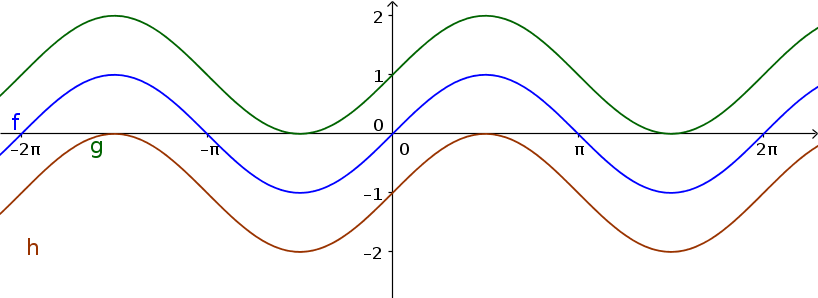Math Sine and cosine function Shifting

# Shifting

## a) along the y-axis

The graphs of $y=\sin(x)$ and $y=\cos(x)$ are shifted with the value $d$ along the y-axis. The general formula is:

$y=\sin(x)+d$
$y=\cos(x)+d$
!

### Remember

• If $d$ > 0, the graph is shifted up.
• If $d$ < 0, the graph is shifted down.

### Example

$\color{green}{g(x)=\sin(x)+1}$
$\color{blue}{f(x)=\sin(x)}$
$\color{brown}{h(x)=\sin(x)-1}$## b) along the x-axis

The graphs of $y=\sin(x)$ and $y=\cos(x)$ are shifted with the value $c$ along the x-axis. The general formula is:

$y=\sin(x+c)$
$y=\cos(x+c)$
!

### Remember

• If $c$ > 0, the graph is shifted to the left.
• If $c$ < 0, the graph is shifted to the right.

### Example

$\color{green}{g(x)=\sin(x+\frac{\pi}{2})}$
$\color{blue}{f(x)=\sin(x)}$
$\color{brown}{h(x)=\sin(x-\frac{\pi}{2})}$